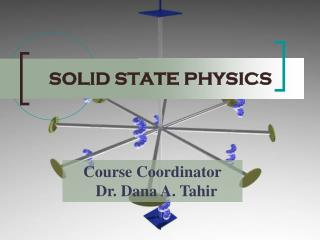Download PresentationSOLID STATE PHYSICS

# SOLID STATE PHYSICS

Download Presentation## SOLID STATE PHYSICS

- - - - - - - - - - - - - - - - - - - - - - - - - - - E N D - - - - - - - - - - - - - - - - - - - - - - - - - - -
##### Presentation Transcript

1. SOLID STATE PHYSICS Course Coordinator Dr. Dana A. Tahir

2. INTRODUCTION • AIM OF SOLID STATE PHYSICS • WHAT IS SOLID STATE PHYSICS AND WHY DO IT? • CONTENT • REFERENCES SOLID STATE PHYSICS INTRODUCTION

3. Aim of Solid State Physics • Solid state physics (SSP) explains the properties of solid materials as found on earth. • The properties are expected to follow from Schrödinger’s eqn. for a collection of atomic nuclei and electrons interacting with electrostatic forces. • The fundamental laws governing the behaviour of solids are known and well tested. SOLID STATE PHYSICS INTRODUCTION

4. Crystalline Solids • We will deal with crystalline solids, that is solids with an atomic structure based on a regular repeated pattern. • Many important solids are crystalline. • More progress has been made in understanding the behaviour of crystalline solids than that of non-crystalline materials since the calculation are easier in crystalline materials. SOLID STATE PHYSICS INTRODUCTION

5. What is solid state physics? • Solid state physics, also known as condensed matter physics, is the study of the behaviour of atoms when they are placed in close proximity to one another. • In fact, condensed matter physics is a much better name, since many of the concepts relevant to solids are also applied to liquids, for example. SOLID STATE PHYSICS INTRODUCTION

6. What is the point? • Understanding the electrical properties of solids is right at the heart of modern society and technology. • The entire computer and electronics industry relies on tuning of a special class of material, the semiconductor, which lies right at the metal-insulator boundary. Solid state physics provide a background to understand what goes on in semiconductors. SOLID STATE PHYSICS INTRODUCTION

7. Solid state physics(SSP) is the applied physics • New technology for the future will inevitably involve developing and understanding new classes of materials. By the end of this course we will see why this is a non-trivial task. • So, SSP is the applied physics associated with technology rather than interesting fundamentals. SOLID STATE PHYSICS INTRODUCTION

8. Electrical resistivity of three states of solid matter • How can this be? After all, they each contain a system of atoms andespecially electrons of similar density.And the plot thickens: graphite is a metal, diamond is an insulatorand buckminster-fullerene is a superconductor. They are all just carbon! SOLID STATE PHYSICS INTRODUCTION

9. Among our aims - understand whyone is a metal and one an insulator,and then the physical origin of themarked features. • Also think about thermal propertiesetc. etc.

10. CONTENT • Chapter 1. Crystal Structure • Chapter 2. X-ray Crystallography • Chapter 3. Interatomic Forces • Chapter 4. Crystal Dynamics • Chapter 5. Free Electron Theory EP364 SOLID STATE PHYSICS INTRODUCTION

11. CHAPTER 1.CRYSTAL STRUCTURE • Elementary Crystallography • Solid materials (crystalline, polycrystalline, amorphous) • Crystallography • Crystal Lattice • Crystal Structure • Types of Lattices • Unit Cell • Directions-Planes-Miller Indices in Cubic Unit Cell • Typical Crystal Structures (3D– 14 Bravais Lattices and the Seven Crystal System) • Elements of Symmetry SOLID STATE PHYSICS INTRODUCTION

12. CHAPTER 2. X-RAY CRYSTALLOGRAPHY • X-ray • Diffraction • Bragg equation • X-ray diffraction methods • Laue Method • Rotating Crystal Method • Powder Method • Neutron & electron diffraction SOLID STATE PHYSICS INTRODUCTION

13. CHAPTER 3. INTERATOMIC FORCES • Energies of Interactions Between Atoms • Ionic bonding • NaCl • Covalent bonding • Comparision of ionic and covalent bonding • Metallic bonding • Van der waals bonding • Hydrogen bonding SOLID STATE PHYSICS INTRODUCTION

14. CHAPTER 4. CRYSTAL DYNAMICS • Sound wave • Lattice vibrations of 1D cystal • Chain of identical atoms • Chain of two types of atoms • Phonons • Heat Capacity • Density of States • Thermal Conduction • Energy of harmonic oscillator • Thermal energy & Lattice Vibrations • Heat Capacity of Lattice vibrations SOLID STATE PHYSICS INTRODUCTION

15. CHAPTER 5. FREE ELECTRON THEORY • Free electron model • Heat capacity of free electron gas • Fermi function, Fermi energy • Fermi dirac distribution function • Transport properties of conduction electrons SOLID STATE PHYSICS INTRODUCTION

16. REFERENCES • Core book: Solid state physics, J.R.Hook and H.E.Hall, Second edition (Wiley) • Other books at a similar level: Solid state physics, Kittel (Wiley) Solid state physics, Blakemore (Cambridge) Fundamentals of solid state physics, Christman (Wiley) • More advanced: Solid state physics, Ashcroft and Mermin SOLID STATE PHYSICS INTRODUCTION